## A gas made up of atoms escapes through a pinhole 0.392 times as fast as Ne gas. Write the chemical formula of the gas.

Question

A gas made up of atoms escapes through a pinhole 0.392 times as fast as Ne gas. Write the chemical formula of the gas.

in progress 0
5 months 2021-08-31T10:31:31+00:00 1 Answers 8 views 0

1. Answer:  The chemical formula of the gas is Xenon.

Explanation:

From Graham’s law of effusion rates, the rate of effusion of a gas is inversely proportional to the square root of its molar mass.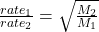Given: Rate of unknown gas =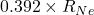Putting the values in the formula: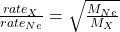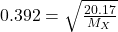Squaring both sides: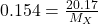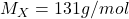As Xenon (Xe) has molar mass of 131g/mol, Thus the chemical formula of the gas is Xenon.